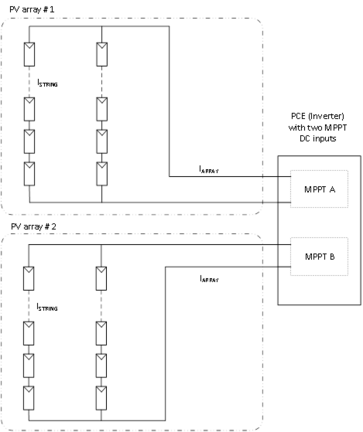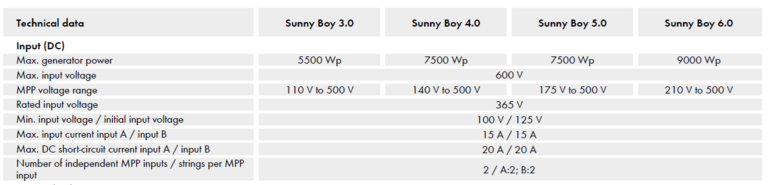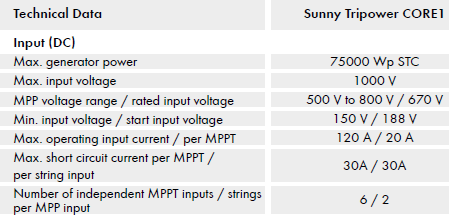# AS/NZS 5033:2021 Array current calculations for SMA inverters

### Summary

On the 20th of May, AS/NZS 5033:2021 became mandatory.  It included new formulas for calculating the maximum current expected from a PV Array.   An inverter must be able to accept this current through its MPPT DC input terminals so it must be considered when selecting a suitable PV module to connect to an inverter MPPT DC input.

### Calculations

The aim is to calculate the maximum array current according to AS/NZS 5033:2021 and compare it to the inverter ISC MPPT rating to confirm the PV array design meets the standards.### Disclaimer

The information contained herein is subject to change. New information will be published as soon as it becomes available.

Last Updated: 05/31/2021### Step 1.

Calculate the maximum string current

It is typical for arrays connected to SMA inverters to be installed without DCU (DC conditioners/optimisers).
AS/NZS 5033:2021 3.3.3 (a) describes how to calculate ISTRING MAX where no DCU are installed.

ISTRING MAX = 1.25 x KI x ISC MOD

Generally, KI has a value of 1 except where bifacial modules are used.
ISC MOD is the STC short circuit current of the PV module in Amps.
It will be available from the PV module datasheet.

### Step 2.

Check backfeed current of inverter MPPT DC input.

ISTRING = ISTRING MAX or IBF TOTAL (whichever is greater)

IBF TOTAL is the backfeed current from sources other than the PV Array.  It can be ignored with the SMA inverters listed in Table 1.  These inverters produce zero backfeed current so IBF TOTAL will always be less than ISTRING MAX.  Backfeed current is termed ‘maximum reverse current’ in SMA inverter manuals.

### Step 3.

Calculate the maximum array current

IARRAY SA x ISTRING MAX

IARRAY is the maximum array current in Amps.
SA is the number of strings in the array.
ISTRING MAX is the maximum current per string in Amps.

### Step 4.

Confirm the maximum array current is less than the inverter MPPT DC input ISC.

## Example 1

Sunny Boy 5kW inverter (SB5.0-1AV-41) to be installed with 16 PV Modules.
Inverter MPPT DC input A has 8 series connected 425W modules, inverter MPPT DC input B has 8 series connected 425W modules.
Modules have an Isc of 11.32A.

ISTRING MAX = 1.25 x KI x ISC MOD

ISTRING MAX = 1.25 x 1 x 11.32A=14.15A

IARRAY = SA x ISTRING MAX

IARRAY = 1x14.15A as there is only one string in the PV array.

If we look at the datasheet for the inverter the maximum short circuit current is 20A.This module is therefore suitable for the inverter MPPT inputs A and B as IARRAY <20A.

## Example 2

Sunny Tripower 50kW inverter (STP50-41) to be installed with 18, 425W PV Modules on each MPPT DC input.
Modules have an Isc of 11.32A.
We will consider one MPPT.

If the 18 modules are connected in one string:

ISTRING MAX = 1.25 x KI x ISC MOD

ISTRING MAX = 1.25 x 1 x 11.32A = 14.15A

ISTRING = ISTRING MAX = IARRAY14.15A as there is only one string in the PV array.

If we look at the datasheet for the inverter the maximum short circuit current is 30A.This module arrangement is therefore suitable for the inverter MPPT DC input as IARRAY <30A.

If the 18 modules are connected in two strings of nine modules:

Modules have an Isc of 11.32A

ISTRING MAX = 1.25 x KI x ISC MOD

ISTRING MAX = 1.25 x 1 x 11.32A = 14.15A

IARRAY = SA x ISTRING MAX

IARRAY = 2 x 14.15A = 28.30A as there are two strings in the PV array.

This module arrangement is therefore suitable for the inverter MPPT DC input as IARRAY <30A.

 Model MPPT Input (labels on inverter) Inv Max Input per MPPT (Amps) Inv Max ISC per MPPT (Amps) PV Module Isc Max 11 string(Amps) PV Module Isc Max 12 strings(Amps) SB1.5-1VL-40 A 10 18 14.4 7.2 SB2.5-1VL-40 A 10 18 14.4 7.2 SB3.0-1AV-41 A,B 15 20 16 8 SB4.0-1AV-41 A,B 15 20 16 8 SB5.0-1AV-41 A,B 15 20 16 8 SB6.0-1AV-41 A,B 15 20 16 8 STP3.0-3AV-40 A,B 12 18 14.4 7.2 STP4.0-3AV-40 A,B 12 18 14.4 7.2 STP5.0-3AV-40 A,B 12 18 14.4 7.2 STP6.0-3AV-40 A,B 12 18 14.4 7.2 STP8.0-3AV-40 A 20 30 24 12 B 12 18 14.4 7.2 STP10.0-3AV-40 A 20 30 24 12 B 12 18 14.4 7.2 STP 50-41 A-F 20 30 24 12 STP 110-60 1-12 26 40 32 16

Table 1

Notes

1IBFTOTAL is equivalent to Maximum Reverse Current described in SMA product manuals.
KI is 1 for this example table but may change with bifacial modules.
‘No DCU’ calculations used in example.